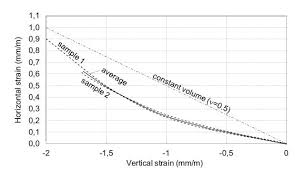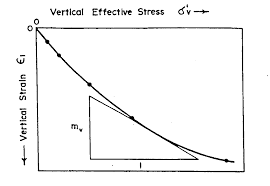## How to Calculate and Solve for Horizontal Strain | Rock MechanicsThe image above represents horizontal strain.

To compute for horizontal strain, five essential parameters are needed and these parameters are Principal Horizontal Stress Component 1 (σH1), Principal Horizontal Stress Component 2 (σH2), Poisson’s Ratio (v), Vertical Stress (σv) and Young’s Modulus (E).

The formula for calculating the horizontal strain:

εH = H1 – vσH2 – vσv) / E

Where:

εH = Horizontal Strain
σH1 = Principal Horizontal Stress Component 1
σH2 = Principal Horizontal Stress Component 2
v = Poisson’s Ratio
σv = Vertical Stress
E = Young’s Modulus

Let’s solve an example;
Find the horizontal strain when the principal horizontal stress component 1 is 7, the principal horizontal component 2 is 9, the Poisson’s ratio is 11, the vertical stress is 21 and young’s modulus is 27.

This implies that;

σH1 = Principal Horizontal Stress Component 1 = 7
σH2 = Principal Horizontal Stress Component 2 = 9
v = Poisson’s Ratio = 11
σv = Vertical Stress = 21
E = Young’s Modulus = 27

εH = H1 – vσH2 – vσv) / E
εH = (7 – 11(9) – 11(21)) / 27
εH = (7 – 99 – 231) / 27
εH = -323 / 27
εH = -11.96

Therefore, the horizontal strain is -11.96.

Calculating the Principal Horizontal Stress Component 1 when the Horizontal Stress, Principal Horizontal Stress Component 2, Poisson’s Ratio, Vertical Stress and Young’s Modulus is Given.

σH1 = (εH x E) + vσH2 + vσv

Where;

σH1 = Principal Horizontal Stress Component 1
εH = Horizontal Strain
σH2 = Principal Horizontal Stress Component 2
v = Poisson’s Ratio
σv = Vertical Stress
E = Young’s Modulus

Let’s solve an example;
Find the principal horizontal stress component 1 when the horizontal strain is 22, the principal horizontal component 2 is 10, the Poisson’s ratio is 7, the vertical stress is 12 and young’s modulus is 3.

This implies that;

εH = Horizontal Strain = 22
σH2 = Principal Horizontal Stress Component 2 = 10
v = Poisson’s Ratio = 7
σv = Vertical Stress = 12
E = Young’s Modulus = 3

σH1 = (εH x E) + vσH2 + vσv
σH1 = (22 x 3) + 7(10) + 7(12)
σH1 = 66 + 70 + 84
σH1 = 220

Therefore, the principal horizontal stress component 1 is 220.

Calculating the Principal Horizontal Stress Component 2 when the Horizontal Stress, Principal Horizontal Stress Component 1, Poisson’s Ratio, Vertical Stress and Young’s Modulus is Given.

σH2 = -(H x E) – σH1 + vσv / v)

Where;

σH2 = Principal Horizontal Stress Component 2
εH = Horizontal Strain
σH1 = Principal Horizontal Stress Component 1
v = Poisson’s Ratio
σv = Vertical Stress
E = Young’s Modulus

Let’s solve an example;
Find the principal horizontal stress component 2 when the horizontal strain is 28, the principal horizontal component 1 is 14, the Poisson’s ratio is 5, the vertical stress is 17 and young’s modulus is 7.

This implies that;

εH = Horizontal Strain =28
σH1 = Principal Horizontal Stress Component 1 = 14
v = Poisson’s Ratio = 5
σv = Vertical Stress = 17
E = Young’s Modulus = 7

σH2 = -(H x E) – σH1 + vσv / v)
σH2 = -((28 x 7) – 14 + 5(17) / 5)
σH2 = -(196 – 14 + 85 / 5)
σH2 = -(182 + 85 / 5)
σH2 = -(267 / 5)
σH2 = -(53.4)
σH2 = -53.4

Therefore, the principal horizontal stress component 2 is -53.4.

## How to Calculate and Solve for Vertical Strain | Rock MechanicsThe image above represents vertical strain.

To compute for vertical strain, five essential parameters are needed and these parameters are Vertical Stress (σv), Poisson’s Ratio (v), Principal Horizontal Stress Component 1 (σH1), Principal Horizontal Stress Component 2 (σH2) and Young’s Modulus (E).

The formula for calculating the vertical strain:

εv = v – vσH1 – vσH2) / E

Where:

εv = Vertical Strain
σv = Vertical Stress
v = Poisson’s Ratio
σH1 = Principal Horizontal Stress Component 1
σH2 = Principal Horizontal Stress Component 2
E = Young’s Modulus

Let’s solve an example;
Find the vertical strain with a vertical stress of 44, Poisson’s ratio of 5, principal horizontal stress component 1 of 6, principal horizontal stress component 2 of 4 and young’s modulus of 12.

This implies that;

σv = Vertical Stress = 44
v = Poisson’s Ratio = 5
σH1 = Principal Horizontal Stress Component 1 = 6
σH2 = Principal Horizontal Stress Component 2 = 4
E = Young’s Modulus = 12

εv = v – vσH1 – vσH2) / E
εv = (44 – 5(6) – 5(4)) / 12
εv = (44 – 30 – 20) / 12
εv = -6 / 12
εv = -0.5

Therefore, the vertical strain is -0.5.

Calculating the Vertical Stress when the Vertical Strain, Poisson’s Ratio, Principal Horizontal Stress Component 1, Principal Horizontal Stress Component 2 and Young Modulus is Given.

σv = (εv x E) + vσH1 + vσH2

Where:

σv = Vertical Stress
εv = Vertical Strain
v = Poisson’s Ratio
σH1 = Principal Horizontal Stress Component 1
σH2 = Principal Horizontal Stress Component 2
E = Young’s Modulus

Let’s solve an example;
Find the vertical stress with a vertical strain of 52, Poisson’s ratio of 12, principal horizontal stress component 1 of 15, principal horizontal stress component 2 of 10 and young’s modulus of 13.

This implies that;

εv = Vertical Strain = 52
v = Poisson’s Ratio = 12
σH1 = Principal Horizontal Stress Component 1 = 15
σH2 = Principal Horizontal Stress Component 2 = 10
E = Young’s Modulus = 13

σv = (εv x E) + vσH1 + vσH2
σv = (52 x 13) + 12(15) + 15(10)
σv = 676 + 180 + 150
σv = 1006

Therefore, the vertical stress is 1006.

Calculating the Poisson’s Ratio when the Vertical Strain, Vertical Stress, Principal Horizontal Stress Component 1, Principal Horizontal Stress Component 2 and Young Modulus is Given.

v = σv – (εv x E) / σH1 + σH2

Where:

v = Poisson’s Ratio
εv = Vertical Strain
σv = Vertical Stress
σH1 = Principal Horizontal Stress Component 1
σH2 = Principal Horizontal Stress Component 2
E = Young’s Modulus

Let’s solve an example;
Find the Poisson’s ratio with a vertical strain of 16, vertical stress of 110, principal horizontal stress component 1 of 7, principal horizontal stress component 2 of 13 and young’s modulus of 6.

This implies that;

εv = Vertical Strain = 16
σv = Vertical Stress = 110
σH1 = Principal Horizontal Stress Component 1 = 7
σH2 = Principal Horizontal Stress Component 2 = 13
E = Young’s Modulus = 6

v = σv – (εv x E) / σH1 + σH2
v = 110 – (16 x 6) / 7 + 13
v = 110 – 96 / 20
v = 14 / 20
v = 0.7

Therefore, the Poisson’s ratio is 0.7.